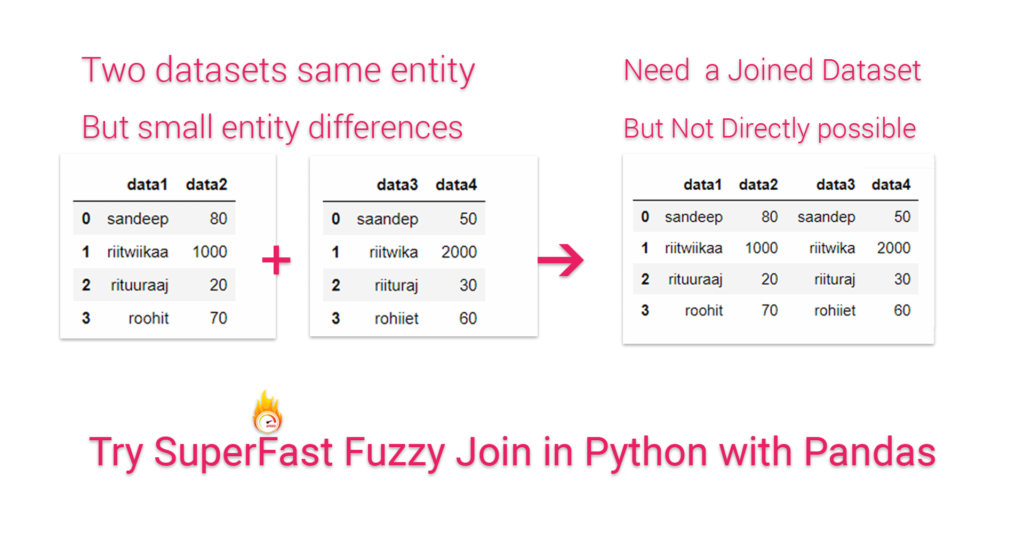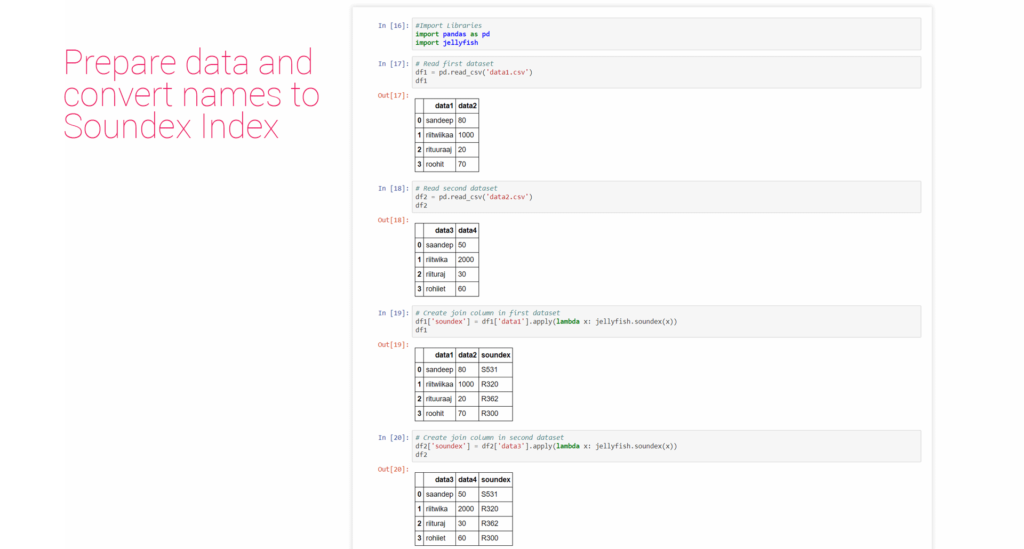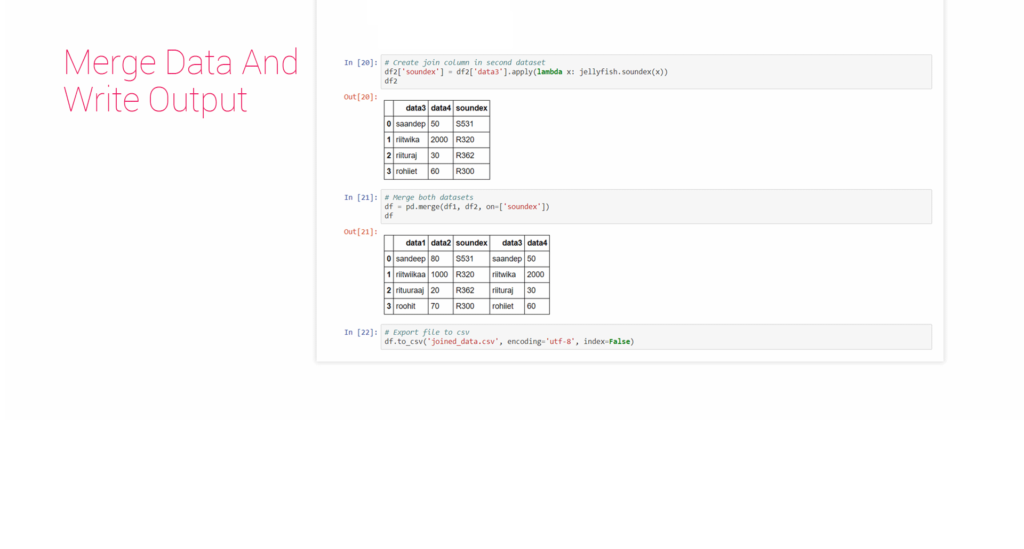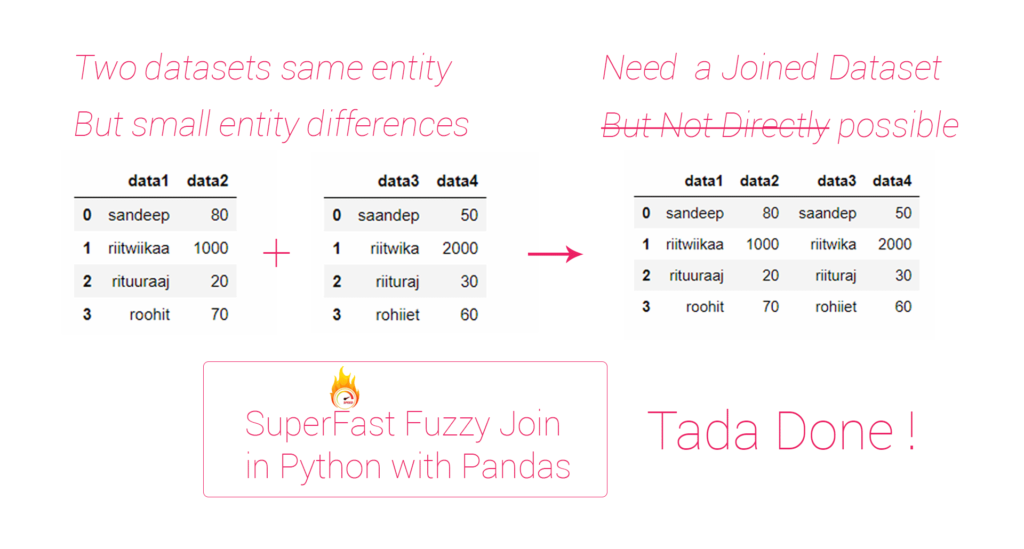## Summary – SUPERFAST FUZZY JOIN IN PYTHON USING PANDAS.

Sometimes we have to join two different data set about same entities but variations in entity name, this makes direct join process incapable of handling the joins and results in mismatch. Here fuzzy join is handy as it uses soundex algorithm  to convert names to the an index based on how it is pronounced.

Pandas is a library in python used by a lot of data scientists, it handles a lot of heavy load in data manipulation.

Jupyter notebook is a python library that provides us an interface where we can code in bits and pieces and see results, and yes it is a bliss for data scientists.

It is recommended to use Anaconda distribution of python, in this tutorial python 3.6 has been used.

Two Steps:

## The ProblemDATASCIENCE-1-2018.11.28-SUPERFAST-FUZZY-JOIN-IN-PYTHON-USING-PANDAS-problem

Problem: Sometimes we have to join two different data set about same entities but variations in entity name, this makes direct join process incapable of handling the joins and results in mismatch..

## Procedure

### Prepare data and convert names to Soundex indexPrepare data and convert names to Soundex index

##### Import libraries:
```#Import Libraries
import panda as pd
import jellyfish
```
```# Read first dataset

# Read second dataset

```
##### Create Soundex Index to join data:
```# Create join column in first dataset
df1['soundex'] = df1['data1'].apply(lambda x: jellyfish.soundex(x))```
```# Create join column in second dataset
df2['soundex'] = df2['data3'].apply(lambda x: jellyfish.soundex(x))

```

Merge data and write output.Merge data and write output.

##### Merge data:
```# Merge both datasets
df = pd.merge(df1, df2, on=['soundex'])```
##### Write output data:
```# Export file to csv
df.to_csv('joined_data.csv', encoding='utf-8', index=False)```